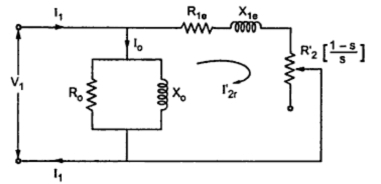### Circle Diagram of a 3 Phase Induction Motor

The equivalent circuit of a 3 phase induction motor is shown in the Fig.1.Fig. 1 Equivalent circuit of a 3 phase induction motor
All the values shown are per phase values. The circuit is similar to  series R-L circuit. The reactance  X1e is fixed while the total resistance R1e + (R2'(1-s)/s) is variable. This is because the slip s varies as load varies. The voltage across the parallel exciting branch is V1. Hence we can write the expression for the rotor current referred to stator as,
I2r' = V1/√((R1e + RL )2 + X1e2)
Where            RL' =  R2' (1-s)/s = Variable equivalent load resistance
R1e = R1 + R2' = Equivalent resistance of motor referred to stator
X1e = X1 + X2' = Equivalent reactance of motor referred to stator
...                             I2r' = Imax sin                                  ....................(1)
where           sinΦ = X/Z = X1e/√((R1e + RL' )2 + X1e2)
and               Imax = V1/X1e
The I2r' will be at its maximum when R1e + RL' = 0 i.e., there exists an ideal short circuit. Hence current Imax is called ideal short circuit current of an induction motor.
The equation (1) represents equation of a circle with as its diameter. Thus locus of extremely of I2r' is a circle, as shown in the Fig.2.Fig. 2 Circle diagram of  I2r'
But the total stator current I1 per phase is a vector addition of current Io and I2r'.
I1 = Io + I2r'                           ....... Vector addition
For and induction motor, Io has a fixed value and phase angle Φo which is decided by its active component and magnetising component Im.
Īo = Īc + Īm
As Io has fixed magnitude and phase, the locus of extremities of I1, which is Io + I2r' is also a circle with a diameter still as V1/X1e. The only charge will be that the diameter V1/X1e will no longer be along X-axis i.e. current axis but will get shifted at the tip of the Io phasor. All the I2r' phasors are to be drawn from Io phasor to get I1, as has fixed magnitude and phase angle Φo.
Key Point : Thus the current locus for a stator current is also a semicircle which is truly called circle diagram of a three phase induction motor. This diagram once obtained can be used to predict the performance of an induction motor under variable load conditions.
The circle diagram is shown in the Fig. 3.Fig. 3  Circle diagram of a three phase induction motor
Let us see, how to obtain the data for plotting the circle diagram.
Obtaining Data to Plot Circle Diagram
The data required to draw the circle diagram is obtained by conducting two testes which are,
1. No load test or open circuit test
2. Blocked rotor test or short circuit test

Related articles :